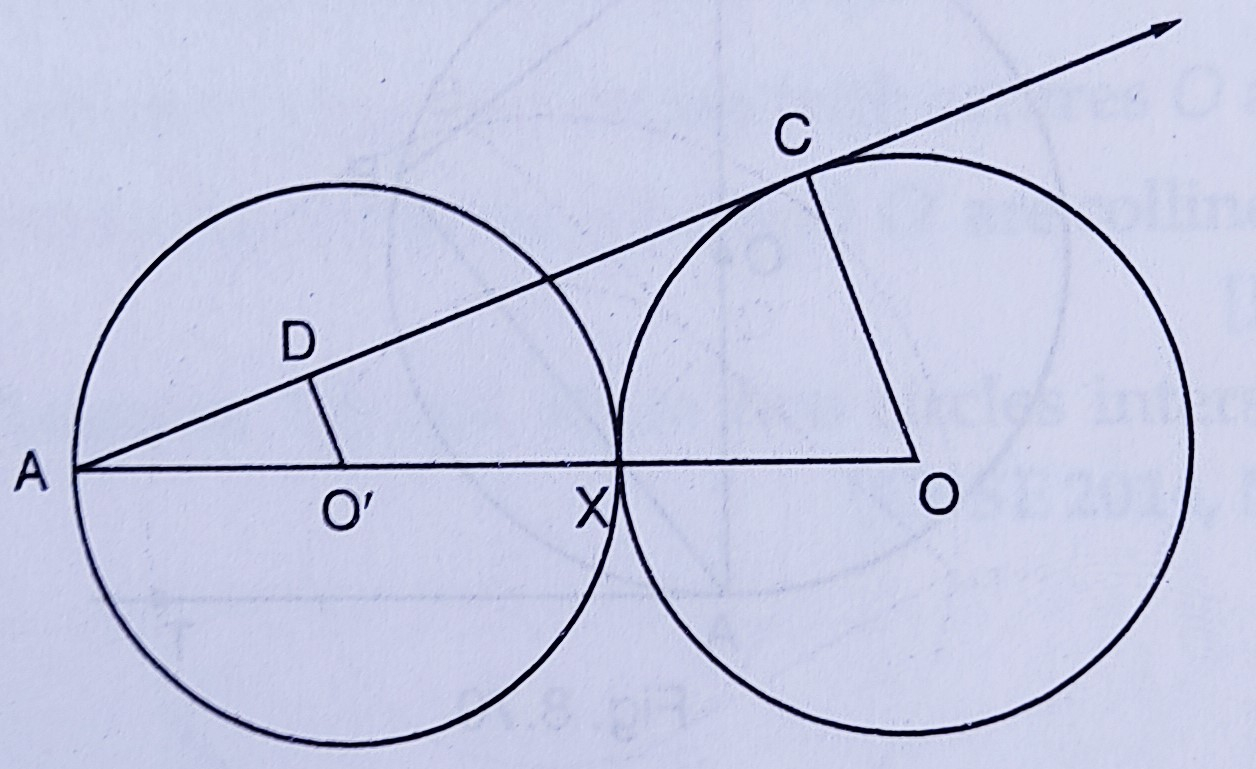"
">

# Equal circles with centres $O$ and $O^{\prime}$ touch each other at $X . O O^{\prime}$ produced to meet a circle with centre $O^{\prime}$, at $A . A C$ is a tangent to the circle whose centre is O. $O^{\prime} D$ is perpendicular to $A C$. Find the value of $\frac{D O^{\prime}}{C O}$."

Given:

Equal circles with centres $O$ and $O^{\prime}$ touch each other at $X . O O^{\prime}$ produced to meet a circle with centre $O^{\prime}$, at $A . A C$ is a tangent to the circle whose centre is O. $O^{\prime} D$ is perpendicular to $A C$.

To do:
We have to find the value of $\frac{D O^{\prime}}{C O}$.

Solution:

$AC$ is the tangent of the circle with centre $O$.

$O^{\prime}D\ perp\ AC$ is drawn and $OC$ is joined.

$AC$ is tangent and $OC$ is the radius.

$OC\ \perp\ AC$

$O^{\prime}D\ \perp\ AC$

$OC\ \parallel\ O^{\prime}D$

$OA=O^{\prime}A+O^{\prime}X+OX$

$OA=3AO^{\prime}$        ($O^{\prime}A=O^{\prime}X=OX$

In triangles $O^{\prime}AD$ and $OAC$,

$\angle A = \angle A$  (Common angle)

$\angle AO^{\prime}D =\angle AOC$    (Corresponding angles)

Therefore,

$\Delta \mathrm{O}^{\prime} \mathrm{AD} \sim \Delta \mathrm{OAC}$     (By AA axiom) $\frac{\mathrm{DO}^{\prime}}{\mathrm{CO}}=\frac{\mathrm{AO}^{\prime}}{\mathrm{AO}}$

$=\frac{\frac{1}{3} \mathrm{AO}}{\mathrm{AO}}$

$=\frac{1}{3}$

The value of $\frac{D O^{\prime}}{C O}$ is $\frac{1}{3}$.

Updated on: 10-Oct-2022

33 Views